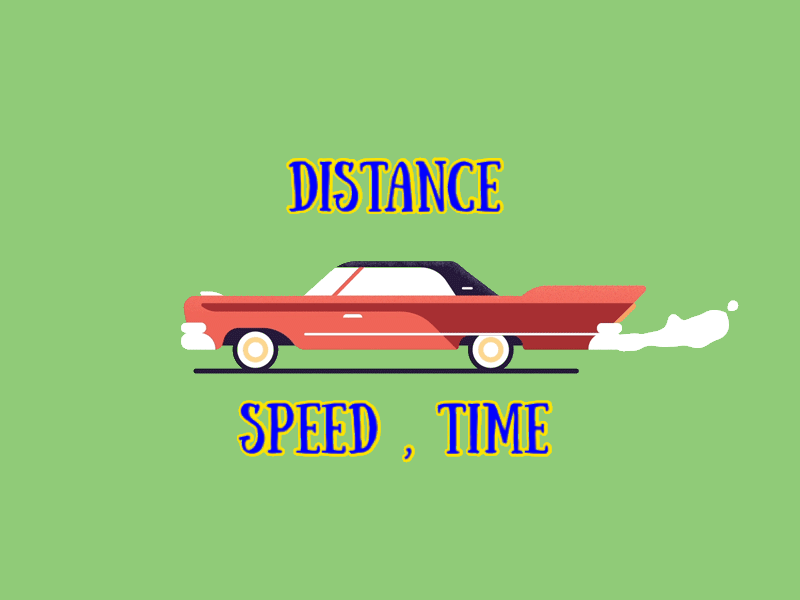Search
•The MA*th Tutor SG

# P6 PSLE Maths (Distance, Speed, Time)

Updated: May 18, 2020Car P left for Town Z from Town Y at 12.15pm. At 2.30pm, Car Q left Town Z for Town Y. Car P takes 73/6 hrs to reach Town Z. Car Q takes 73/8 hrs to reach Town Y. Car P would have travelled 75 km more than Car Q when both of them met.

a)What was the distance between the 2 towns?

b) What was the speed of Car P and Car Q, respectively?

Car P left for Town Z from Town Y at 12.15pm. At 2.30pm, Car Q left Town Z for Town Y. Car P takes 73/6 hrs to reach Town Z. Car Q takes 73/8 hrs to reach Town Y. Car P would have travelled 75 km more than Car Q when both of them met. a)What was the distance between the 2 towns? b) What was the speed of Car P and Car Q, respectively? ➡️In 1hr, Car P travelled = 1 / (73/6) = 6/73 of YZ Car Q travelled = 1 / (73/8) = 8/73 of ZY Total distance covered in 1hr= 14/73 ➡️From 12.15 pm to 2.30pm Total no of hrs= 2.25hrs

Before Car Q set off for Town Y,Car P would have travelled = 2.25 x (6/73)= 27/146 of YZ (Car P) At 2.30pm, Distance from Town Z= 1- (27/146)= 119/146 ➡️Time taken to meet from 2.30pm onwards= (119/146) ➗ (14/73) = 4.25hrs ➡️Total time Car P would have travelled before meeting Car Q = 4.25 + 2.25 = 6.5hrs ➡️Total time for Car Q would have travelled before meeting Car P = 4.25hrs ➡️(Car Q)Distance Travelled in 4.25hrs = 4.25 x (8/73) = 34/73 ➡️(car P) Distance travelled in 6.5hrs= 6.5x (6/73)= 39/73 ➡️Car P would have travelled a more distance of = (39/73) - (34/73) = 5/73 In other words 5u= 75km 1u= 15km 73u= 1095km (a) YZ= 1095km (b) Speed of Car P= 1095/(73/6) = 90km/h Speed of Car Q= 1095/(73/8) =120km/h

Website: www.scholastictuitionsg.com

[Prepare Your Child For The Upcoming PSLE Exams]

Over the years, the Primary School Math syllabus has undergone changes. Most notably, the syllabus places more emphasis on developing students’ problem-solving abilities and and thinking skills when they are doing questions which require them to ‘think out of the box’.

Rote learning (memorizing by constantly doing the same practice over and over again till perfection) may not be sufficient in order to ACE that Math Exams!

Hence, today, the honing of one’s ability to see and approach 1 question from many perspectives, SUPPLEMENTED BY concepts from several topics AS WELL AS trying out creative problem solving methods to answer that particular question is the norm.

We at The Math Tutor SG want to introduce students, via our FB Page: fb.me/TheMathTutorSG, to a wide range of questions, which not only test students on the fundamental concepts (numerical, geometrical, algebraic, statistical, probabilistic and analytical) but also stretch their thinking when they attempt our questions across the topics of whole numbers, fractions, decimals, percentages, ratios, speed, area and volume!

We hope that through our sample questions, students can develop their skills in Reasoning, the Heuristics Processes and Thinking, Numerical Calculation, Algebraic Manipulation, Spatial Visualization, Data Analysis, Measurement and Estimation, inculcate the belief, confidence and interest as well as develop an appreciation for Math. Equally as important, through our questions, students are able to monitor their own thinking.